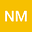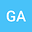Analytical study of the chiral nonlinear Schr\”{o}dinger’s equation for optical Soliton Solutions
••The aim of the present paper is to extract the exact traveling wave solutions of the chiral nonlinear Schr\”{o}dinger’s equation (cNLSE). The $(\frac{G’}{G^{2}})-$expansion method and the first integral method along with symbolic computation package has been exerted to celebrate the exact solutions. As a consequence, the obtained solutions can be categorized into trigonometric, hyperbolic and rational with some free parameters of the problem studied. In addition, these types of the solutions lead to understand the physical phenomena of the problem such as solitary, periodic, complex function, singular optical solitons and dark-singular combo solitons.